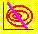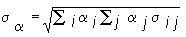6.7 THREE-FIRM CASE: CML

The objective is to find the line with the largest slope connecting the risk-free asset with points on the minimum-variance frontier. This line will be tangent to the minimum-variance frontier. You can apply CAPM Tutor to find this point of tangency and see the CML using the default data set. If you change the risk-free interest rate, you will see how the point of tangency and the slope of the CML also change.

We start by considering three lines that intersect the frontier. These are portfolios having different target returns:

 Target Return Portfolio I: 0.158 Portfolio II: 0.159 Portfolio III: 0.160

You learned the method for determining the portfolio weights that correspond to a particular target return E in the Three-Firm Case. The equations for the portfolio weights as a function of E are:

Firm 1 = 6.288E - 0.460

Firm 2 = -1.153E + 0.338

Firm 3 = -5.075E + 1.122

Substituting our three targets, 0.158, 0.159, and 0.160, for E yields the results in Table 6.3.

 Table 6.3 Target Returns and Portfolio Weights

 Portfolio I Portfolio II Portfolio III Target Expected Return 0.158 0.159 0.160 Firm 1 weight 0.524 0.530 0.536 Firm 2 weight 0.156 0.155 0.154 Firm 3 weight 0.320 0.315 0.310

To calculate the riskiness of each portfolio, recall the variance-covariance matrix for the Three-Firm Case:

 0.961 0.063 -0.582 0.063 0.928 -0.359 -0.582 -0.359 0.727

The diagonal terms are the security return variances for securities 1, 2, and 3, respectively. The off-diagonal terms are the covariances. For example, the variance of return for security 2 is 0.928 and the covariance between securities 1 and 2 is 0.063.

The standard deviation of the portfolios can be calculated using the formulaThe resulting standard deviations for Portfolios I, II, and III are:

 Table 6.4 Portfolio Standard Deviations and Slopes

 Portfolio I Portfolio II Portfolio III Target Expected Return 0.158 0.159 0.160 Standard Deviation 0.328 0.337 0.346 Slope 0.115751 0.115755 0.115752

Finally, the slope of the line between the risk-free security and the portfolio is computed by taking the vertical rise over the horizontal run. The vertical rise is the difference between the target return and the risk-free rate. The horizontal run is the portfolio's standard deviation (because the standard deviation for the risk-free security is zero).

Using CAPM Tutor you can verify that this slope of Portfolio I is 0.115751, as in Table 6.4.

The maximum slope is attained by the Portfolio II, with a target return equal to 0.159. This is actually the point of tangency between the capital market line and the efficient frontier. Therefore, the line passing through the risk-free rate and Portfolio II represents the capital market line for the Three-Firm Case.

The CML describes a linear risk/return trade-off for the market as a whole, but what type of trade-off is implied for individual securities? This question is answered in topic 6.8, titled Security Market Line.

previous topic

next topic